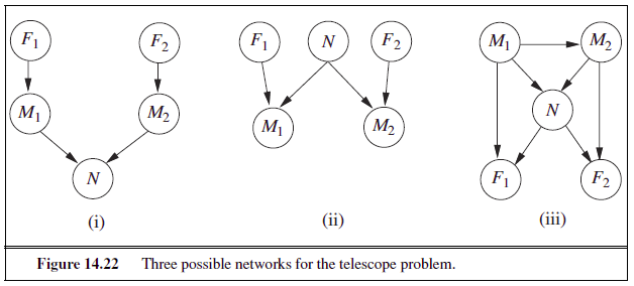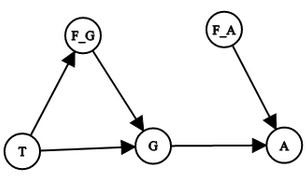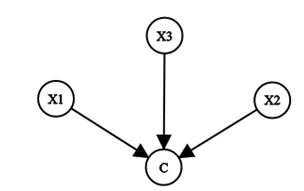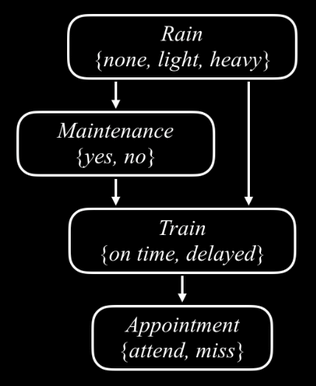# Quiz: Probabilistic reasoning & Bayesian networks¶Consider the Bayesian network in the figure above. In the Conditional Probability Tables (CPTs), the letters B, E, A, J and M stand for Burglary, Earthquake, Alarm, JohnCalls, and MaryCalls, respectively.

True or False: If no evidence is observed, Burglary and Earthquake are independent.Consider the Bayesian network in the figure above. In the Conditional Probability Tables (CPTs), the letters B, E, A, J and M stand for Burglary, Earthquake, Alarm, JohnCalls, and MaryCalls, respectively.

True or False: If we observer Alarm = true, Burglary and Earthquake are independent.Two astronomers in different parts of the world make measurements M 1 and M 2 of the number of stars N in some small region of the sky, using their telescopes. Normally, there is a small possibility e of error by up to one star in each direction. Each telescope can also (with a much smaller probability f) be badly out of focus (events F 1 and F 2), in which case the scientist will undercount by three or more stars (or if N is less than 3, fail to detect any stars at all). Consider the three networks shown in the figure above.

Which of these Bayesian networks are correct (but not necessarily efficient) representations of the preceding information?Two astronomers in different parts of the world make measurements M 1 and M 2 of the number of stars N in some small region of the sky, using their telescopes. Normally, there is a small possibility e of error by up to one star in each direction. Each telescope can also (with a much smaller probability f) be badly out of focus (events F 1 and F 2), in which case the scientist will undercount by three or more stars (or if N is less than 3, fail to detect any stars at all). Consider the three networks shown in the figure above.

Which is the best network?Consider the Bayesian network shown in the figure above. All variables are Boolean, and B=BrokeElectionLaw, I=Indicted, M=PoliticallyMotivatedProsecutor, G=FoundGuilty, J=Jailed.

Which of the following are asserted by the network structure?

i. P(B,I,M) = P(B)P(I)P(M)

ii. P(J|G) = P(J|G,I)Consider the Bayesian network shown in the figure above. All variables are Boolean, and B=BrokeElectionLaw, I=Indicted, M=PoliticallyMotivatedProsecutor, G=FoundGuilty, J=Jailed.

Calculate the value of P(b, i, ¬m, g, j)

Your answers will be evaluated to 4 decimal places. Use a period as a decimal separator.Consider the Bayesian network shown in the figure above. All variables are Boolean, and B=BrokeElectionLaw, I=Indicted, M=PoliticallyMotivatedProsecutor, G=FoundGuilty, J=Jailed. Calculate the probability that someone goes to jail given that they broke the law, have been indicted, and face a politically motivated prosecutor. Your answers will be evaluated to 4 decimal places. Use a period as a decimal separator.

Let H x be a random variable denoting the handedness of an individual x, with possible values l or r. A common hypothesis is that left- or right-handedness is inherited by a simple mechanism; that is, perhaps there is a gene G x, also with values l or r, and perhaps actual handedness turns out mostly the same (with some probability s) as the gene an individual possesses. Furthermore, perhaps the gene itself is equally likely to be inherited from either of an individual’s parents, with a small non-zero probability m of a random mutation flipping the handedness. The following figure shows three possible structures for a Bayesian network describing genetic inheritance of handedness:Which of the three networks in the figure claim that P(G father, G mother, G child) = P(G father )P(G mother )P(G child)?

Let H x be a random variable denoting the handedness of an individual x, with possible values l or r. A common hypothesis is that left- or right-handedness is inherited by a simple mechanism; that is, perhaps there is a gene G x, also with values l or r, and perhaps actual handedness turns out mostly the same (with some probability s) as the gene an individual possesses. Furthermore, perhaps the gene itself is equally likely to be inherited from either of an individual’s parents, with a small non-zero probability m of a random mutation flipping the handedness. The following figure shows three possible structures for a Bayesian network describing genetic inheritance of handedness:Which of the three networks make independence claims that are consistent with the hypothesis about the inheritance of handedness?

Let H x be a random variable denoting the handedness of an individual x, with possible values l or r. A common hypothesis is that left- or right-handedness is inherited by a simple mechanism; that is, perhaps there is a gene G x, also with values l or r, and perhaps actual handedness turns out mostly the same (with some probability s) as the gene an individual possesses. Furthermore, perhaps the gene itself is equally likely to be inherited from either of an individual’s parents, with a small non-zero probability m of a random mutation flipping the handedness. The following figure shows three possible structures for a Bayesian network describing genetic inheritance of handedness:Which of the three networks is the best description of the hypothesis?

Let H x be a random variable denoting the handedness of an individual x, with possible values l or r. A common hypothesis is that left- or right-handedness is inherited by a simple mechanism; that is, perhaps there is a gene G x, also with values l or r, and perhaps actual handedness turns out mostly the same (with some probability s) as the gene an individual possesses. Furthermore, perhaps the gene itself is equally likely to be inherited from either of an individual’s parents, with a small non-zero probability m of a random mutation flipping the handedness. The following figure shows three possible structures for a Bayesian network describing genetic inheritance of handedness:Consider the following table. Suppose that P(G father = l) = P(G mother = l) = 0.5. In the same network, what will P(G child = l) be if we take m = 0.1, by conditioning on its parent nodes.Your answers will be evaluated to 1 decimal places. Use a period as a decimal separator.

In your local nuclear power station, there is an alarm that senses when a temperature gauge exceeds a given threshold. The gauge measures the temperature of the core. Consider the Boolean variables A (alarm sounds), F A (alarm is faulty), and F G (gauge is faulty), as well as the multivalued nodes G (gauge reading) and T (actual core temperature).

Given that the gauge is more likely to fail when the core temperature gets too high, which of the following best represents the Bayesian network for this domain:

1.2.3.In your local nuclear power station, there is an alarm that senses when a temperature gauge exceeds a given threshold. The gauge measures the temperature of the core. Consider the Boolean variables A (alarm sounds), F A (alarm is faulty), and F G (gauge is faulty), as well as the multivalued nodes G (gauge reading) and T (actual core temperature).

Suppose there are just two possible actual and measured temperatures, normal and high; the probability that the gauge gives the correct temperature is x when it is working, but y when it is faulty. Fill the conditional probability table associated with G. Let x = 0.8, y = 0.1. What is b:Your answers will be evaluated to 1 decimal places. Use a period as a decimal separator.

In your local nuclear power station, there is an alarm that senses when a temperature gauge exceeds a given threshold. The gauge measures the temperature of the core. Consider the Boolean variables A (alarm sounds), F A (alarm is faulty), and F G (gauge is faulty), as well as the multivalued nodes G (gauge reading) and T (actual core temperature).

Suppose there are just two possible actual and measured temperatures, normal and high; the probability that the gauge gives the correct temperature is x when it is working, but y when it is faulty. Fill the conditional probability table associated with G. Let x = 0.8, y = 0.1. What is c:Your answers will be evaluated to 1 decimal places. Use a period as a decimal separator.

We have a bag of three biased coins a, b, and c with probabilities of coming up heads of 20%, 60%, and 80%, respectively. One coin is drawn randomly from the bag (with equal likelihood of drawing each of the three coins), and then the coin is flipped three times to generate the outcomes X 1, X 2, and X 3. Take the random variable C to denote which coin {a,b,c} we drew.

Which of the following is the Bayesian network corresponding to this setup?

1.2.3.We have a bag of three biased coins a, b, and c with probabilities of coming up heads of 20%, 60%, and 80%, respectively. One coin is drawn randomly from the bag (with equal likelihood of drawing each of the three coins), and then the coin is flipped three times to generate the outcomes X 1, X 2, and X 3. Take the random variable C to denote which coin {a,b,c} we drew.

Calculate which coin was most likely to have been drawn from the bag if the observed flips come out heads twice and tails once.

Consider the following Bayesian network:Which of the following statements is true?

Posting submission...